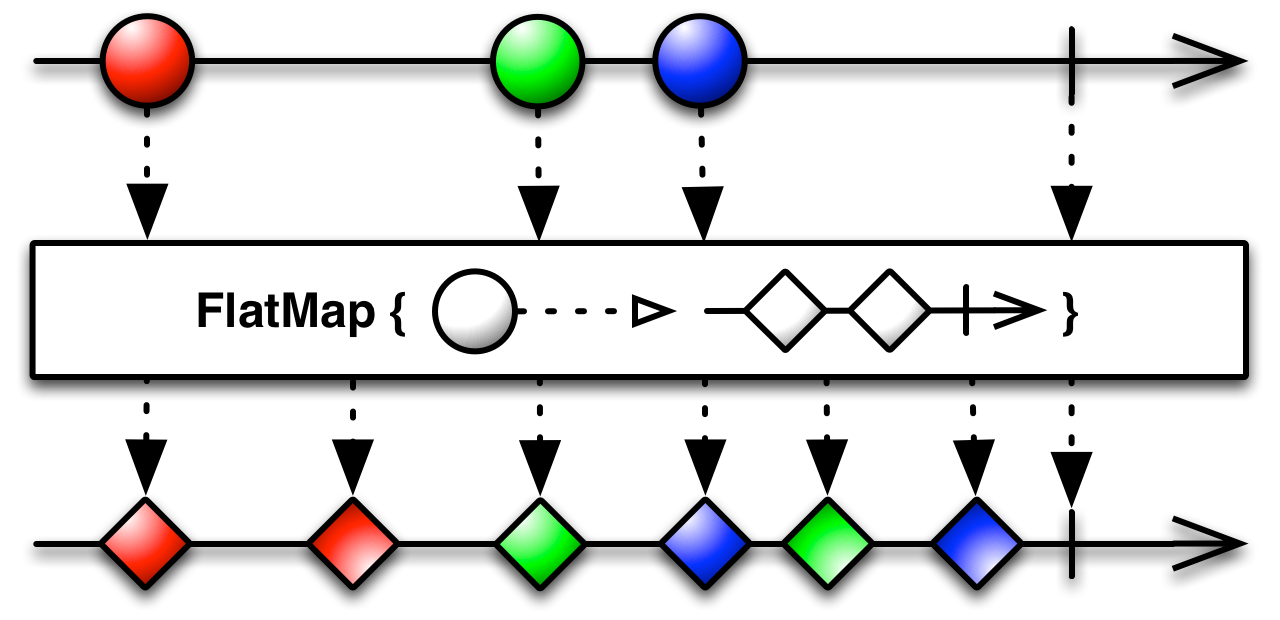# What the FlatMap?

Understand flatmap in RxJavaThe operator `flatmap` in RxJava is a tough topic if you are not familiar with functional style.

After reading all the articles and tutorials and even worked with RxJava for almost a year, I’m still not quite confident about it. I sure know how to and when to use it, but the understanding seems always shallow.

Until I read the `flatmap` in the Structure and Interpretation of Computer Programs.

The key is to understand the word flat.

# FlatMap in the SICP book

Let me give the context when the flatmap is introduced in the SICP book.

First let’s define the function `accumulate`.

``````(define (accumulate op init seq)
(if (null? seq)
init
(op (car seq) (accumulate op init (cdr seq)))))
``````

This is a very simple procedure that generate one value out of a sequence, by using some `operation`.

For example:

``````(accumulate + 0 (list 1 2 3 4))

=> 10
``````

Now we have everything ready to define the `flatmap` procedure.

``````(define (flatmap proc seq)
(accumulate append '() (map proc seq)))
``````

`proc` is a procedure that returns a sequence.

`append` just concats two lists together like

``````(append (list 1 2 3) (list 4 5 6))

=> (1 2 3 4 5 6)
``````

We can describe the process of `flatmap` as follows:

``````;; the original list
(1 2 3)

;; map to a new list of lists
((1 1 1) (2 2 2) (3 3 3))

;; flatten out
(1 1 1 2 2 2 3 3 3)
``````

# FlatMap in RxJava

You can think of the streams in RxJava as lists in SICP. Just streams feels more dynamic, and lists feels more static. The concept is now much easier to understand.

Since streams and lists are very similar, we can apply the same idea to streams.

``````;; the original stream
<1, 2, 3, end>

;; map to a new stream of streams
<<1,1,1,end>, <2,2,2,end>, <3,3,3,end>>

;; flatten out
<1, 1, 1, 2, 2, 2, 3, 3, 3, end>
``````

Note that one item in the original stream 1, is mapped to a new stream `<1, 1, 1, end>`.

Even though this is what flatmap really means, it is not the most common usecase.

The most common usecase is a special case of it, that is to map the item in the original stream to a new stream of one item.

``````;; the original stream
<1, 2, 3, end>

;; map to a new stream of streams
<<a, end>, <b, end>, <3, end>>

;; flatten out
<a, b, c, end>
``````

This is where people got it confused with `map`, because from the outside look it seems that we map the `<1, 2, 3, end>` to `<a, b, c, end>`, but they are totally different processes.

One thing to be careful is the ordering of the items in the flattened stream, since streams emits items asynchronously, when flattened out, the ordering is not garanteed if we use `flatmap` in RxJava. So to be techniquely correct, in the above example we should use `concatmap`.